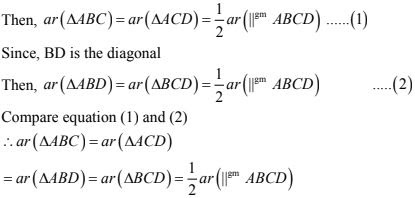# Chapter 15 Areas of Parallelograms and Triangles RD Sharma Solutions Exercise 15.2 Class 9 Maths

 Chapter Name RD Sharma Chapter 15 Areas of Parallelograms and Triangles Exercise 15.2 Book Name RD Sharma Mathematics for Class 10 Other Exercises Exercise 15.1Exercise 15.3 Related Study NCERT Solutions for Class 10 Maths

### Exercise 15.2 Solutions

1. In fig below, ABCD is a parallelogram, AE ⊥ DC and CF ⊥ AD. If AB  = 16 cm, AE = 8 cm and CF = 10cm, find AD.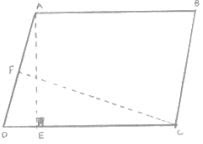Solution

Given that,
In a parallelogram ABCD, CD = AB = 16cm [Opposite sides of a parallelogram are equal]
We know that,
Area of parallelogram = base × corresponding attitude
Area of parallelogram ABCD = CD × AE = AD × CF
16 cm × 8 cm  = AD × 10 cm
AD = (16 × 8)/10 cm  = 12.8 cm
Thus, the length of AD is 12.8 cm

2. In Q. No 1, if AD  = 6 cm, CF = 10 cm, and AE = 8cm, find AB.

Solution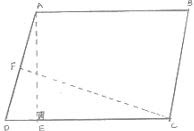We know that,
Area of parallelogram ABCD = AD × CF ...(1)
Again area of parallelogram ABCD = DC × AE  ...(2)
Compare equation (1) and equation (2)
AD × CF = DC × AE
⇒ 6 × 10 = D × B
⇒ D = 60/8 = 7.5 cm
∴ AB = DC = 7.5 cm  [∴ Opposite sides of a parallelogram are equal]

3. Let ABCD be a parallelogram of area 124 cm2. If E and F are the mid - points of sides AB and CD respectively, then find the area of parallelogram AEFD.

Solution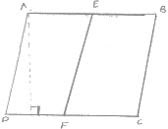Given,
Area of parallelogram ABCD = 124 cm2
Construction : draw AP ⊥ DC
Proof:
Area of parallelogram AFED = DF × AP
And area of parallelogram EBCF = FC × AP
And DF = FC ...(3)  [F is the midpoint of DC]
Compare equation (1), (2) and (3)
Area of parallelogram AEFD = Area of parallelogram EBCF
∴ Area of parallelogram AEFD = (Area of parallelogram ABCD)/2
= 124/2 = 62 cm2 .

4. If ABCD is a parallelogram, then prove that
ar(ΔABD) = ar(ΔBCD) = ar(ΔABC) = ar(ΔACD) = 1/2ar (|| gm ABCD)

Solution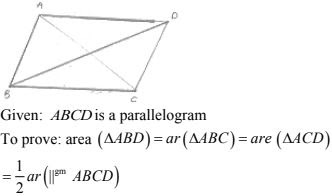Proof: we know that diagonals of a parallelogram divides it into two equilaterals.
Since, AC is the diagonal .